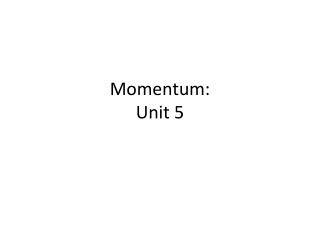DownloadDownload PresentationMomentum: Unit 5

# Momentum: Unit 5

Télécharger la présentation## Momentum: Unit 5

- - - - - - - - - - - - - - - - - - - - - - - - - - - E N D - - - - - - - - - - - - - - - - - - - - - - - - - - -
##### Presentation Transcript

1. Momentum:Unit 5

2. What is Momentum? • Momentum: ______ in motion • The more _______ an object has, the more ________ there is possible. • ________ affects the momentum of an object. • EX. A fast car can have more momentum than a slow truck w/ greater mass • Non-moving objects have _______________ at all.

3. How do we calculate momentum • Momentum= _____________ • p = m x v • Unit: ____________

4. Which has more momentum • A Baseball thrown by a major league pitcher at 90 mph • A dump truck moving at 35 mph

5. Momentum • Changing the mass changes the momentum

6. Momentum • Changing the ________ also changes the momentum • **Remember a change in velocity is called ____________ • Accelerations are produced by __________. • The greater the force= Greater an object’s change in velocity = Greater change in momentum.

7. Impulse • The quantity ________________ = Impulse • F(∆ t) = Impulse • The greater the impulse on an object, the greater the change in momentum • Impulse = _________________ • Impulse = Ft = ∆(mv) • t is the time interval (∆ t) • Unit = N x s

8. Increasing Momentum • Impulse = Impact Force x time measured in ________________

9. Decreasing Momentum • The same impulse decreases your momentum whether hitting a wall or haystack

10. Does this mean the same force? • No!! • The same impulse _____________ with the same force or time. • The same impulse means the product of the force and time ____________

11. Impact time is the key • ____________ is extended when you hit the haystack rather than the wall, THUS the overall impact force is spread out over a longer time. • Impact time = the time during which your momentum is brought to ______. • The longer the impact time = reduction in force of impact = reduction in acceleration

12. Examples • What are some examples of things that increase impact time and therefore decrease force? • Airbags • Padded dashboard v. rigid dashboard • Bending your knees when you jump from an elevated position • Relaxing your body when you fall to spread force of impact over more muscles • Falling on a soft mat vs. hard floor • Moving away from or with a punch • EX. Increasing the time of impact 100 times reduces force of impact 100 times. • _____ and _________ are indirectly proportional.(Two variables that affect impulse)

13. Examples Boxer vs. martial artist

14. Bouncing • Impulses are greater when an object bounces. • Why? The impulse lasts twice as long • 1. time to reduce the momentum to zero. • 2. time to make the object bounce up again.

15. Pelton Water Wheel • Lester A. Pelton designed a water wheel that had curved blades that caused the water to bounce and make a U-turn. • Why do you think Pelton designed his water wheel like this?

16. Pelton Water Wheel • By guiding the water into a u-turn, Pelton got a much greater ________. • Like when a ball bounces

17. Conservation of momentum • Circus physics video

18. Conservation of Momentum • The Law of _____________________: In the absence of an external force, the momentum of a system remains unchanged.

19. Conservation of Momentum • To accelerate an object, a _______ must be applied to the object. • To change the momentum of an object, you must exert an ________ on it. • If no net force (no net impulse) acts on a system, the momentum of that system cannot change.

20. Elastic Collisions • _____________: When objects collide without being permanently deformed and without generating heat. (Bouncing) • Perfectly elastic collisions are not common in the everyday world- some heat is produced in the collisions.

21. Inelastic Collisions • ______________: objects collide and become tangled or coupled together generating heat and becoming distorted in the process. (Sticking) • Most collisions usually involve some external force.